<title> <META NAME="description" CONTENT="AquaCalc LLC / JBS Instruments specializes in stream flow measurement devices like the AquaCalc that are used in the field of hydrology."> <META NAME="keywords" CONTENT="jbs instruments aquacalc aquacount stream flow measurement water discharge current meter hydrology usgs"> </title>

# Using Non-Standard Current Meters including OTT type and Redback current meters

The AquaCalc has the current meter rating equations for many standard United States Geological Survey (USGS) meters built in when shipped to the user. The AquaCalc can also accept custom current meter equations for non-standard USGS and European type horizontal axis meters. Most contact type current meters can be used with the AquaCalc if the rating equation is known from calibration tests.

In addition to the built-in current meter rating curves for the Price AA and Pygmy meters, the AquaCalc can store rating curves for user defined “non-standard” current meters including OTT type meters and Hydrological Services Redback Price AA type current meter.

Please See the “Meter Setup” section in the AquaCalc Pro Plus Instruction Manual for more information onthe use of, and setting up current meters in the AquaCalc Pro Plus. The manual can be downloaded here.

Other information can be entered for each current meter in the AquaCalc using the first page of the Meters screen including:

• 1)Name: (Your name for th current meter i.e. : PriceAA
• 2)ID #: (ID or serial number for current meter)
• 3)Type: NonStd (Select “NonStd” for entering a NonStandard current meter rating)
• 4)Units: (SAE or METRIC)
• 5)Revs/Pulses (Revolutions per Pulse)
• 6)Min.Depth (Minimum Depth for meter)
• 7)Max.Depth (Maximum Depth for meter use)
• 8)Min.Velocity (Minimum Velocity for meter use)
• 9)Max.Velocity (Minimum Velocity for meter use)

As used by the USGS, meter rating curves are either a single or pair of equations that define the relationship between the number of revolutions per second (counts divided by time) of the current meter cups and the measured velocity. These equations are use to create the meter rating tables used in manual measurements.

Non-standard current meter ratings can be defined in several segments, one of which is used for lower velocities, the other segments for progressively higher velocities. Each segment is represented by an equation. A crossover or “breakpoint” velocity value is also specified that indicates the velocity at which the next equation is used.

Each equation takes the form:

y = m * R + b

where R is the Revolutions per second

or

Velocity = m * (revolutions/second) + b

Where m is the slope of the line and b is the value where the line intercepts the velocity axis (which in the AquaCalc is identified as the “Offset”).

Velocity = Slope * (revolutions/second) + Offset

The AquaCalc can handle non-standard meter rating curves with up to three line segments representing three velocity ranges. The “Intercept” value in the AquaCalc represents the crossover or breakpoint velocity value at which second (or third) segment is used.

Figure : Current Meter Definition in the AquaCalc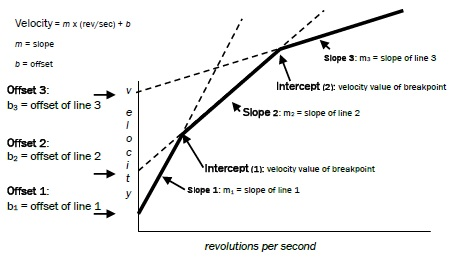## Step-by-step procedure to add a Non-Standard current meter to an AquaCalc Pro Plus

1. From the AquaCalc Main Menu, select number 4) Meters
2. Use the right arrow navigation key to select a new meter to specify.
3. Press number 1) Name: to name this meter
4. Press number 2) ID #: to enter the meter ID number
5. Press number 3) Type: to select the type of meter which should be set to “NonStd” or Non-Standard.
6. Press number 4) Units: to change to “METRIC” if required.
7. Press number 5) Revs/Pulses to change revolutions/pulses (1/1 is standard)
8. Using the “More” Soft-Key, go to the next page where meter rating values are entered.
9. Utilizing keypad number 3 through 0, enter meter constants from the current meter rating equation.
10. Press Save to save the entered values

Be sure to test and confirm the meter and meter ratings before using in the field.

## Example Entry of a Two Part Current Meter Rating Equation

So for a sample Price Type AA Standard No. 1 two segment equation example (This rating is no longer used and has been replaced with new Price Type AA Standard No. 2 below and is included as an example only):

EQUATIONS: V=2.18R + .020(2.200) 2.17R + .030

Note that the value in parenthesis (2.200) represents the velocity above which the second segment is used. The above equation would be entered into the second AquaCalc Meter Settings screen as follows: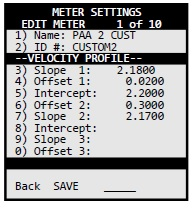## Example of a Single Segment (linear) Current Meter Rating

A single segment rating curve equation (such as the new Price Type AA Standard No. 2) would be entered in the second Meter Setup screen as:

EQUATION: V = 2.2048R + 0.0178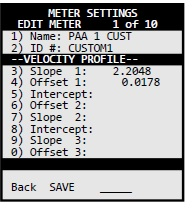## Custom Current Meter Rating for a Hydrological Services Redback Price AA style current meter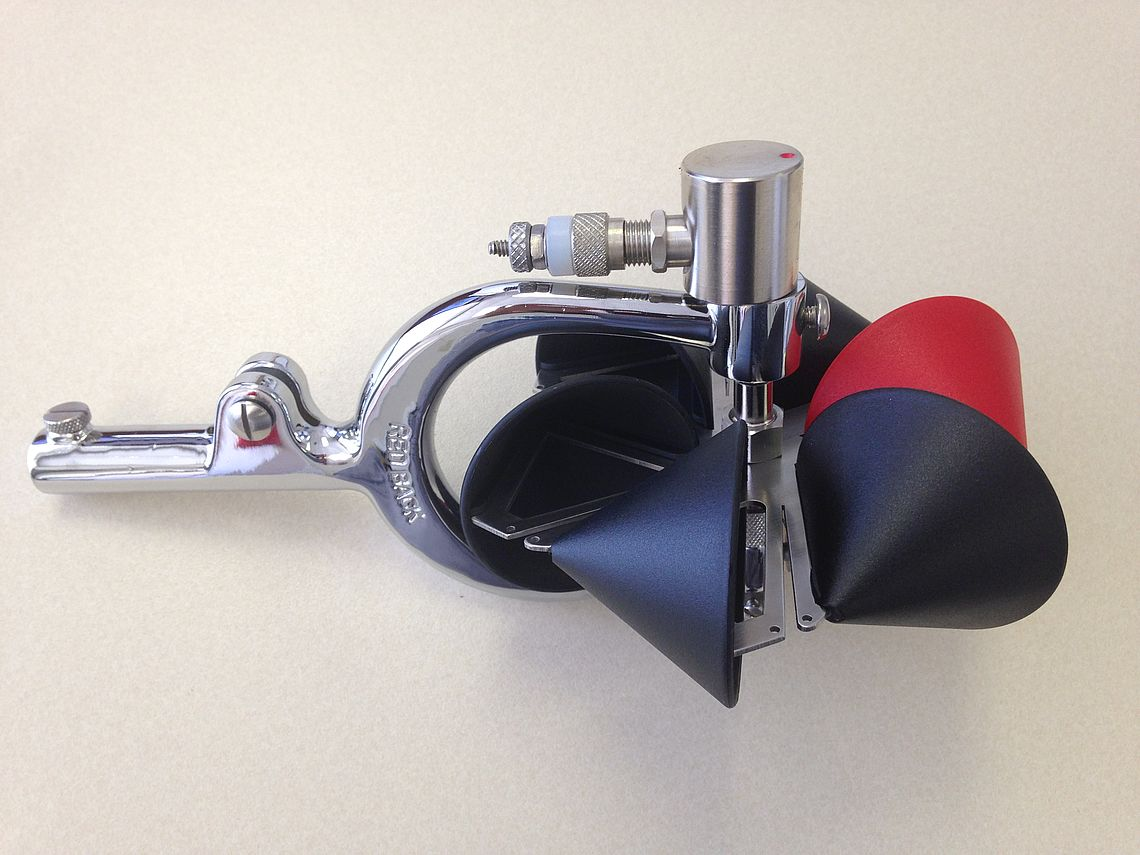Until AquaCalc LLC implements the Hydrological Services Redback current meter as one of the standard ratings in the AquaCalc , existing AquaCalc Pro Plus (and Pro) users can enter the following values into any available “Non-Standard” custom meter in the Meters screen. Once entered, the custom meter is stored and available any time. (Note: In the AquaCalc meter setup screen, the velocity crossover is labeled as “Intercept”):

Hydrological Services has provided the following equations for the current meter rating for a Redback current meter:

n <= 0.14      V = 2.1535 n +0.0308 ft/s

0.14 < n < 2.69        V = 2.2904 n +0.0115 ft/s

n >= 2.69       V = 2.3481 n -0.1437 ft/s

Multipart USGS current meter ratings use a crossover velocity instead of the n = revolutions per second crossover point, but these are easily converted. From the table attached to your rating, the 0.14 rev/sec is equal to 0.332 ft/sec, and the 2.69 rev/sec is equal to 6.173 ft/sec.

The USGS traditionally uses a specific format for multipart ratings,  V= Slope1 * R + Offset1 (VC) Slope2 * R + Offset2, Where R is revolutions per second, and VC is the velocity at the crossover point.

Translating the Redback “Imperial” rating into the USGS format yields: V

V=2.1535R + 0.0308 (0.332) 2.2904R + 0.0115 (6.173) 2.3481R - 0.1437

And this is entered as a Non-Standard (NonStd) Meter Type into the second page of the Meter screen in the AquaCalc Pro Plus as:

### Meter Screen Page 1:

• 1)Name: REDBACK
• 2)ID #: 0-00E
• 3)Type: NonStd
• 4)Units: SAE
• 5)Revs/Pulses 1/1
• 6)Min.Depth (Minimum Depth for meter is unknown)
• 7)Max.Depth (Maximum Depth for meter use is unknown)
• 8)Min.Velocity (Minimum Velocity for meter use is unknown)
• 9)Max.Velocity (Minimum Velocity for meter use is unknown)

### Meter Screen Page 2:

• Name: REDBACK
• ID #: 0-00E (Or as needed)
• -VELOCITY PROFILE-
• Slope 1:     2.1535
• Offset 1:    0.0308
• Intercept 1: 0.332
• Slope 2:     2.2904
• Offset 2:    0.0115
• Intercept 2: 6.173
• Slope 3:     2.3481
• Offset 3:  - 0.1437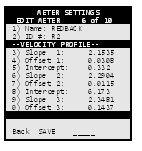## Custom OTT type Horizontal Axis Current Meter Ratings

Horizontal Axis Current Meters that use a contact closure, like the OTT horizontal axis current meter, and the Rickly Hydrological Universal Current Meter - Model 6500, can be used with the AquaCalc Pro Plus by entering a Non-Standard Meteras discussed above, and using the current meter rating equation provided by the manufacturer. This type of current meter uses different “propeller” sizes. Each propeller size has its own rating equation, and must be entered as new and different Non-Standard Meter in the AquaCalc.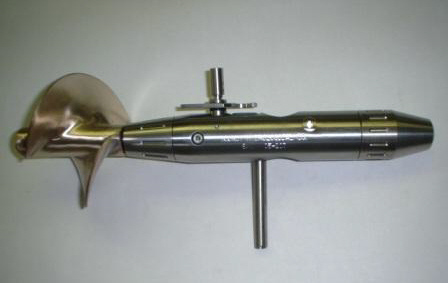For example: The Rickly Hydrological Universal Current Meter with the ”A” type prop has a metric (meters per second) rating equation of:

V=0.1234R + 0.0212 (Note: This is a Metric Rating and should be used with the Metric setting in the AquaCalc)

And this is entered as a “Non-Standard” Meter Type (NonStd) in the first page of the Meter screen with the following values in the second page :

### Meter Screen Page 1:

• 1)Name: UNIV A
• 2)ID #: 9999
• 3)Type: NonStd
• 4)Units: METRIC
• 5)Revs/Pulses 1/1
• 6)Min.Depth (Minimum Depth for meter)
• 7)Max.Depth (Maximum Depth for meter use)
• 8)Min.Velocity (Minimum Velocity for meter use)
• 9)Max.Velocity (Minimum Velocity for meter use)

### Meter Screen Page 2:

• Name: UNIV A
• ID #: 0-00E
• -VELOCITY PROFILE-
• Slope 1:     0.1234
• Offset 1:    0.0212
• Intercept 1:
• Slope 2:
• Offset 2:
• Intercept 2:
• Slope 3:
• Offset 3:

Using Custom Current Meters with the AquaCalc

AquaCalc LLC. · telephone +1.916.372.0534 · e-mail: sales@aquacalc.com

## Need Accessories or Cables?

We have replacement parts and cables for the AquaCalc line of stream flow computers including the Digital Pygmy Magnetic Head retro fit your old Pygmy Current Meter with a new digital magnetic sensor.

Find Accessories and Cables here.